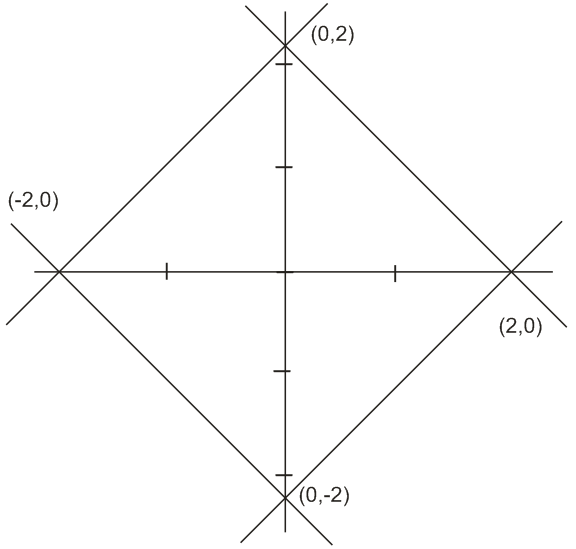# The region represented by  and  is bounded by a : Option 1) square of side length  units Option 2) rhombus of side length 2 units Option 3) square of area 16 sq. units Option 4) rhombus of area  sq. units

region bounded by  and

Graph of this isArea = 8

side =

option (1) is correct.

Option 1)

square of side length  units

Option 2)

rhombus of side length 2 units

Option 3)

square of area 16 sq. units

Option 4)

rhombus of area  sq. units

Exams
Articles
Questions